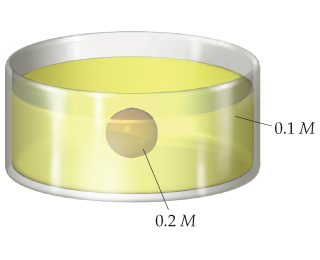# Problem: Suppose you had a balloon made of some highly flexible semipermeable membrane. The balloon is filled completely with a 0.2 M solution of some solute and is submerged in a 0.1 M solution of the same solute:Initially, the volume of solution in the balloon is 0.25 L.Assuming the volume outside the semipermeable membrane is large, as the illustration shows, what would you expect for the solution volume inside the balloon once the system has come to equilibrium through osmosis?

###### FREE Expert Solution

In this problem, we are asked what would we expect for the solution volume inside the ballon once the system is in equilibrium through osmosis.

Recall that osmosis is the flow of solvent from a solution of lower solute concentration to one of higher concentration.

• the pressure requires to stop osmosis is called the osmotic pressure

To solve this problem, we must look at the principles that govern osmosis.

83% (445 ratings)###### Problem Details

Suppose you had a balloon made of some highly flexible semipermeable membrane. The balloon is filled completely with a 0.2 M solution of some solute and is submerged in a 0.1 M solution of the same solute:
Initially, the volume of solution in the balloon is 0.25 L.Assuming the volume outside the semipermeable membrane is large, as the illustration shows, what would you expect for the solution volume inside the balloon once the system has come to equilibrium through osmosis?